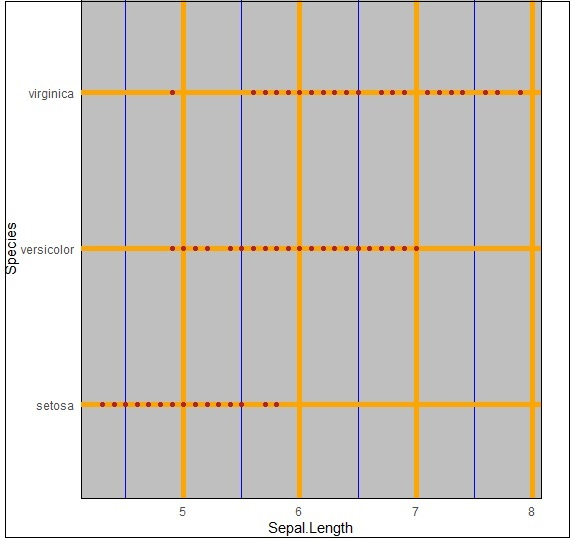# ggplot2 - Background Colors

There are ways to change the entire look of your plot with one function as mentioned below. But if you want to simply change the background color of the panel you can, use the following −

## Implementing Panel background

We can change the background color using following command which helps in changing the panel (panel.background) −

```> ggplot(iris, aes(Sepal.Length, Species))+geom_point(color="firebrick")+
+ theme(panel.background = element_rect(fill = 'grey75'))
```

The change in color is clearly depicted in picture below −## Implementing Panel.grid.major

We can change the grid lines using property “panel.grid.major” as mentioned in command below −

```> ggplot(iris, aes(Sepal.Length, Species))+geom_point(color="firebrick")+
+    theme(panel.background = element_rect(fill = 'grey75'),
+    panel.grid.major = element_line(colour = "orange", size=2),
+    panel.grid.minor = element_line(colour = "blue"))
```We can even change the plot background especially excluding the panel using “plot.background” property as mentioned below −

```ggplot(iris, aes(Sepal.Length, Species))+geom_point(color="firebrick")+
+   theme(plot.background = element_rect(fill = 'pink'))
```